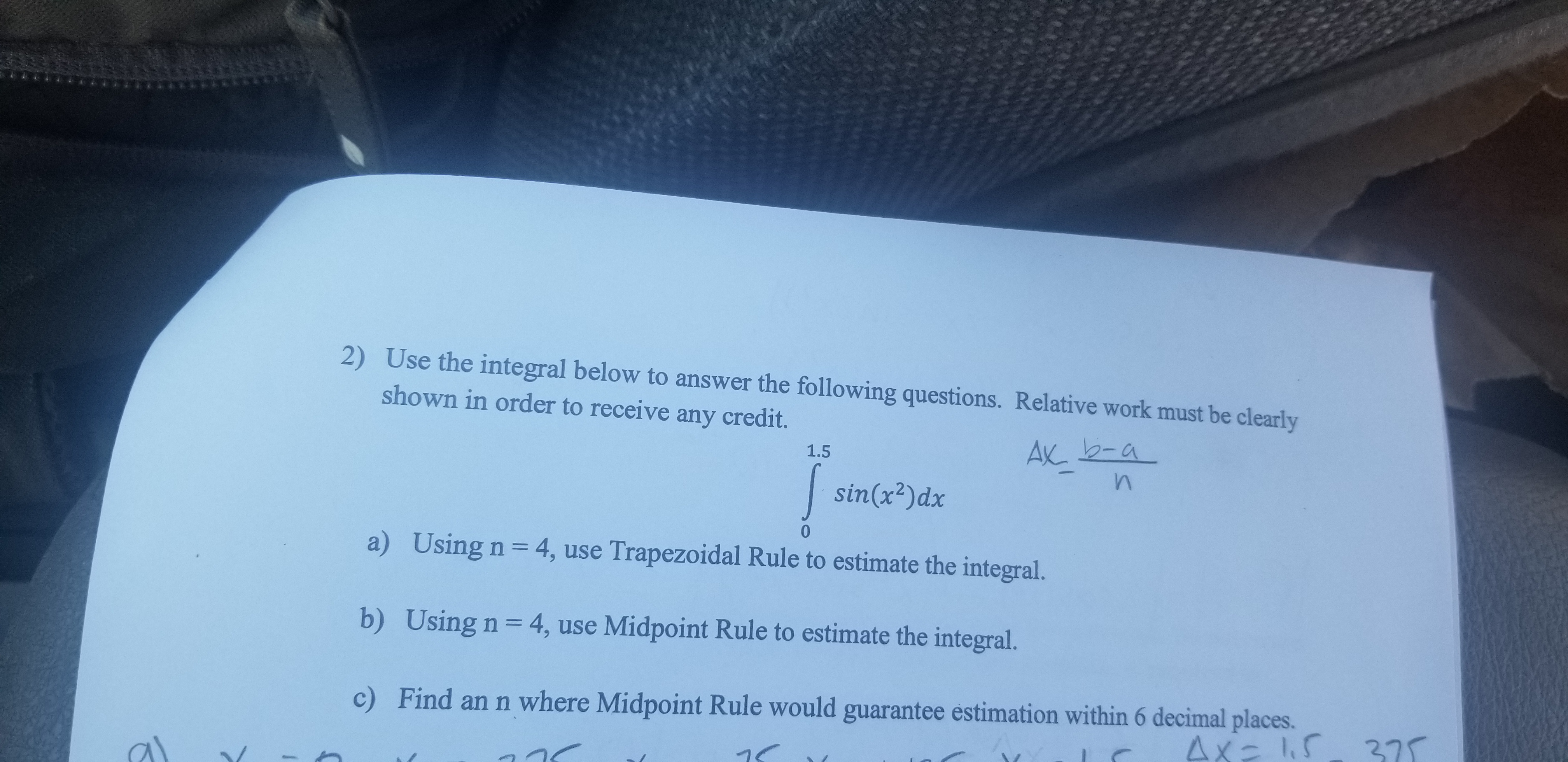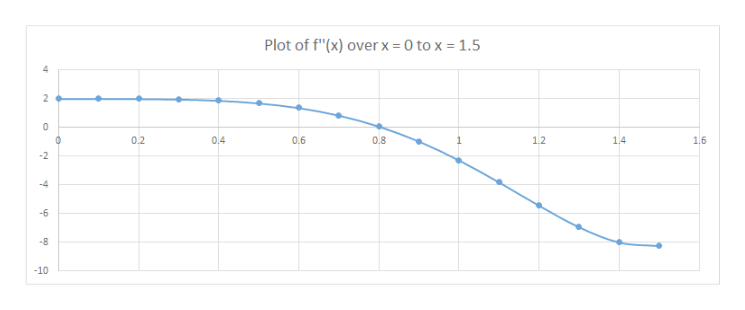# 2) Use the integral below to answer the following questions. Relative work must be clearlyshown in order to receive any credit.AX 2-a1.5sin(x2)dxa)Using n = 4, use Trapezoidal Rule to estimate the integral.b) Using n 4, use Midpoint Rule to estimate the integral.Find an n where Midpoint Rule would guarantee estimation within 6 decimal places.c)4x- 15 27

Question
65 views

C). Find an 'n' where Midpoint Rule would guarantee estimation within 6 decimal places.help_outlineImage Transcriptionclose2) Use the integral below to answer the following questions. Relative work must be clearly shown in order to receive any credit. AX 2-a 1.5 sin(x2)dx a) Using n = 4, use Trapezoidal Rule to estimate the integral. b) Using n 4, use Midpoint Rule to estimate the integral. Find an n where Midpoint Rule would guarantee estimation within 6 decimal places. c) 4x- 15 27 fullscreen
check_circle

Step 1

Let's get the concepts in place, before we proceed to solve this.

For an integral I (as shown on the white board), the error EM under mid point rule is as given by the expression on the whiteboard.

“h” used in the expression has been defined further.

“n” is what we have to find such that EM ≤ 10-6

f(x) = sin(x2)dx

a = 0, b = 1.5

Step 2

We now proceed to calculate the double derivative of f(x). Recall

• the chain rule of calculus
• d(sinz)/dz = cosz
• : d(xn) / dx = nxn-1

Step 3

In order to get the maximum value of f''(x), we need to plot f''(x) w.r.t over x = 0 to x = 1.5.

Please see the graph on the white board.

The maximum absolu...help_outlineImage TranscriptionclosePlot of f"(x) overx 0 to x 1.5 2 0 0.2 0.4 0.6 0.8 1.2 1.4 1.6 R -10 fullscreen

### Want to see the full answer?

See Solution

#### Want to see this answer and more?

Solutions are written by subject experts who are available 24/7. Questions are typically answered within 1 hour.*

See Solution
*Response times may vary by subject and question.
Tagged in

### Derivative